login  home  contents  what's new  discussion  bug reports help  links  subscribe  changes  refresh  edit

 Submitted by : (unknown) at: 2007-11-17T22:06:46-08:00 (15 years ago) Name : Axiom Version : default friCAS-20090114 Axiom-20050901 OpenAxiom-20091012 OpenAxiom-20110220 OpenAxiom-Release-141 Category : Axiom Aldor Interface Axiom Compiler Axiom Library Axiom Interpreter Axiom Documentation Axiom User Interface building Axiom from source lisp system MathAction Doyen CD Reduce Axiom on Windows Axiom on Linux Severity : critical serious normal minor wishlist Status : open closed rejected not reproducible fix proposed fixed somewhere duplicate need more info Optional subject :   Optional comment :

Axiom doesn't seem to do the integral of sin(x^2), but both Maxima and Mathematica (per http://integrals.wolfram.com/ anyway) produce answers. Confirmed by Martin Rubey, and uploaded at his request to IssueTracker.

CY

fricas
integrate(sin(x^2),x)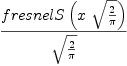(1)
Type: Union(Expression(Integer),...)

However:

 Maxima 5.9.1.1cvs http://maxima.sourceforge.net
Using Lisp CMU Common Lisp 19b (19B)
Dedicated to the memory of William Schelter.
This is a development version of Maxima. The function bug_report()
provides bug reporting information.
(%i1) integrate(sin(x**2),x);
(sqrt(2) %i + sqrt(2)) x
(%o1) sqrt(%pi) ((sqrt(2) %i + sqrt(2)) erf(------------------------)
2
(sqrt(2) %i - sqrt(2)) x
+ (sqrt(2) %i - sqrt(2)) erf(------------------------))/8
2
(%i2)



It's worth noting that both Maple and Mathematica produce the FresnelS? function, which is defined in terms of this integral. However, the Maxima answer appears to be correct as well.

Note that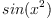is a D-finite function:

fricas
f := sin(x^2)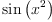(2)
Type: Expression(Integer)
fricas
4*x^3*f - D(f,x) + x*D(f,x,2)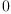(3)
Type: Expression(Integer)

Thus integration should be "easy"...

I browsed the web a little more and came to the conclusion that the Risch algorithm only deals with elementary functions whose integral is elementary, too. "Clearly" (looking at maxima's output or browsing the web),does not have an elementary antiderivative. Hence, I suspect that it should be treated just as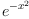, by the PatternMatchIntegration package. This would not be too difficult, probably.

Martin

Although this does not really solve the original problem, I think I found a bug in INTPM. Currently, there is an operation:
           pmComplexintegrate(f, x) ==
(rc := splitConstant(f, x)).const ^= 1 =>
(u := pmintegrate(rc.nconst, x)) case "failed" => "failed"
rec := u::ANS
[rc.const * rec.special, rc.const * rec.integrand]
cse := (rec := matcherfei(f, x, true)).which
cse = ERF  => [rec.coeff * erf(rec.exponent), 0]
"failed"


It is pretty obvious that the third line should read:

               (u := pmComplexintegrate(rc.nconst, x)) case "failed" => "failed"


fricas
complexIntegrate(-%i/2*e^(%i*x^2),x)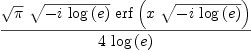(4)
Type: Expression(Complex(Integer))

fricas
complexIntegrate(%i/2*e^(-%i*x^2),x)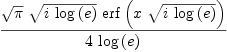(5)
Type: Expression(Complex(Integer))

For some reason, it still won't do

fricas
complexIntegrate(sin(x^2),x)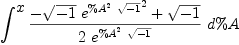(6)
Type: Expression(Integer)

Curiously, the pattern matcher is not even invoked in this case... Even if we enter the integral as

fricas
complexIntegrate(-%i/2*e^(%i*x^2)+%i/2*e^(-%i*x^2),x)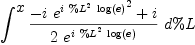(7)
Type: Expression(Complex(Integer))

it fails, although in this case the pattern matcher is invoked. It would need to be invoked on each summand seperately, though.

Martin

There is another issue I don't quite understand. Currently axiom returns the whole integral unevaluated if it does not manage to evaluate it completely. If it were not for the bug #199, the following were an example:
fricas
integrate(exp(x)/x+exp(-x^2)+1/log(x),x)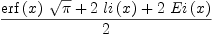(8)
Type: Union(Expression(Integer),...)

Although axiom produces the intermediate result integrate(exp(x)/x+exp(-x^2),x)+li(x), it discards it. The responsible line of code is in rinteg$FSINT :  rinteg(i, f, x, h, comp) == not elem? i => integral(f, x)$F
empty? rest(l := [mkPrimh(f, x, h, comp) for f in expand i]) => first l
l


Does this make sense?

Martin

partially fixed in FriCAS

Status: open => closed

 Subject:   Be Bold !! ( 14 subscribers )